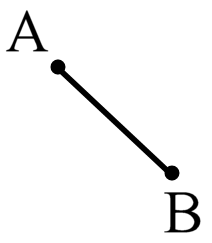20 Questions | Total Attempts: 1701SettingsMathematics grade 7 quiz is a simple set of questions, which have been designed to help all the students in 7th grade to revise and better their mathematics skills. If you are one of them, take it up. All the best.

• 1.
Coplanar lines that do not meet?
• A.

Parallel lines

• B.

Intersecting lines

• C.

Perpendicular lines

• D.

Skew lines

• 2.
An angle formed by an intersecting lines that are supplementary and are next to each other?
• A.

Vertical angles

• B.

• C.

Complementary Angle

• D.

Supplementary Angle

• 3.
These two lines do not lie on a plane?
• A.

Skew line

• B.

Coplanar

• C.

Parallel line

• D.

Collinear

• 4.
It is a part of a line that has two endpoints?
• A.

Ray

• B.

Segment

• C.

Number line

• D.

Line segment

• 5.
Lines that intersect at a right angle?
• A.

Skew line

• B.

Perpendicular line

• C.

Vertical line

• D.

Intersecting line

• 6.
These are points on the same line?
• A.

Noncollinear Points

• B.

Line

• C.

Ray

• D.

Collinear

• 7.
An angle formed by an intersecting lines that are on the diagonals and have the same measure?
• A.

Corresponding

• B.

Supplementary Angle

• C.

Vertical Angle

• D.

• 8.
These are points that are on the same plane?
• A.

Coplanar Points

• B.

Line Points

• C.

Collinear Points

• D.

Nonecollinear Points

• 9.
It has no dimension?
• A.

Angle

• B.

Point

• C.

Plane

• D.

Line

• 10.
What do you call an angle whose measure is 90° ?
• A.

Right Angle

• B.

Acute Angle

• C.

Supplementary Angle

• D.

• 11.
How many lines can be drawn passing through a point?
• A.

Only one

• B.

Only two

• C.

Infinite

• D.

None of the above

• 12.
How many lines in a plane are said to be concurrent if all of therm intersect at the same point?
• A.

Only two

• B.

Three or More

• C.

Only three

• D.

None of the above

• 13.
If a line can be drawn through a set of points, then the points are called ______ points.
• A.

Coplanar

• B.

Collinear

• C.

Vertical

• D.

Noncollinear

• 14.
Two rays that both start from a common point and go off in exactly opposite directions
• A.

Infrared rays

• B.

Line

• C.

Opposite rays

• D.

Ray

• 15.
Which of the following best describes vertical angles?
• A.

They don't have to be next to each other, just so long as the total is 180 degrees.

• B.

They don't have to point in the same direction.

• C.

The same vertex and one side

• D.

Opposite angles formed by intersecting lines

• 16.
How would you label AB?
• A.

Line

• B.

Line Segment

• C.

Ray

• D.

Angle

• 17.
An angle measures less than 90 degrees.
• A.

Acute angle

• B.

• C.

Obtuse ngle

• D.

Right angle

• 18.
What angle was shown below?
• A.

Complementary angle

• B.

• C.

Right angle

• D.

Vertical angle

• 19.
The figure below is a_____.
• A.

Coplanar

• B.

Letter line

• C.

Collinear line

• D.

Line segment

• 20.
Which of the following describe an adjacent angle?
• A.

Three or more lines in a plane

• B.

An angle have a common side and a common vertex

• C.

Each of the pairs of opposite angles made by two intersecting lines.

• D.

All of the above

Related TopicsBack to top